﻿ 背景误差水平相关结构对四维变分资料同化的影响研究
 气象学报2020, Vol. 78Issue (6): 988-1001PDF
http://dx.doi.org/10.11676/qxxb2020.062

0

#### 文章信息

GONG Jiandong, ZHANG Lin, WANG Jincheng. 2020.

An impact study of background error horizontal correlation structure on 4DVar

Acta Meteorologica Sinica, 78(6): 988-1001.
http://dx.doi.org/10.11676/qxxb2020.062

### 文章历史

2020-02-03 收稿
2020-07-06 改回

An impact study of background error horizontal correlation structure on 4DVar
GONG Jiandong, ZHANG Lin , WANG Jincheng
National Meteorological Center/Numerical Weather Prediction Center，Beijing 100081，China
Abstract: Temporal evolution characteristics of the GRAPES global 4DVar analysis increment in the spectral space is investigated, and the impact on the analysis and forecast is also analyzed when the background error horizontal correlation characteristics at the beginning and end of the data assimilation time window are obviously different. Three types of horizontal correlation model, i.e., the second-order autoregressive model (SOAR), the statistical model from the samples generated by ensemble of data assimilation (EDA), and the blending results of SOAR and EDA, are compared. The results show that the information of synoptic scale in the analysis increment is obviously underestimated by the SOAR model. For the horizontal correlation power spectrum calculated by blending of the SOAR and the EDA, the results show a multi-scale horizontal correlation characteristics, which can better absorb observation information and significantly improve the forecast of geopotential height and temperature in the northern hemisphere. The wind forecast is also improved in the southern hemisphere, while a neutral impact is found in the tropical region. The above results indicate that the merging horizontal correlation scheme developed in this paper is reasonable and practical.
Key words: GRAPES 4DVar    Background error horizontal correlation    Variance spectra blending    Numerical experiment
1 引　言

2 4DVar分析增量的功率谱分布特征 2.1 公式表达及误差特征分析

GRAPES全球4DVar对模式初值的最优估计可以表述为求解以下目标函数的极小值（Zhang，et al，2019

 $\begin{split} J({\text δ}{{X}}^{\mathrm{a}})=&\frac{\,1\,}{\,2\,}{{\text δ}{{X}}^{\mathrm{aT}}}{{B}}_{0}^{-1}{\text δ}{{X}}^{\mathrm{a}}+\frac{\,1\,}{\,2\,}\sum\limits_{{i}=0}^{{n}}\Big[{({{d}}_{{i}}\!-\!{{H}}_{{i}}{{M}}_{0,{i}}{\text δ}{{X}}^{\mathrm{a}})}^{\mathrm{T}}{\text •}\\&{{R}}^{-1}({{d}}_{{i}}\!-\!{{H}}_{{i}}{{M}}_{0,{i}}{\text δ}{{X}}^{\mathrm{a}})\Big]+\!{J}_{\mathrm{c}} \end{split}$ (1)

4DVar中采用分析变量的变量变换方式来避免显式求解背景误差协方差矩阵（ ${{B}}_{0}$ ）的逆矩阵（张华等，2004王瑞春等，2015）。利用根号矩阵表达 ${{B}}_{0}={{B}}_{0}^{1/2}{{B}}_{0}^{\mathrm{T}/2}$ ，以及变量变换 ${\text δ}{{X}}^{\mathrm{a}}={{B}}_{0}^{1/2}{v}$ ${v}$ 为控制变量，是归一化的无量纲向量，在GRAPES全球4DVar中， ${v}=({\psi },{{\chi }}_{\mathrm{u}},{{\pi }}_{\mathrm{u}},{q})$ ，分别为流函数、非平衡势函数、非平衡无量纲气压以及比湿场。根号矩阵（ ${{B}}_{0}^{1/2}$ ）用误差模型近似表达为 ${{B}}_{0}^{1/2}={P}{{\varSigma }}_{\mathrm{u}}{U}$ ，其中 ${P}$ 为物理变换，主要实现非平衡物理变量向物理变量的转换以及从流函数与势函数到风场的物理变换， ${{\varSigma }}_{\mathrm{u}}$ 为非平衡部分的背景误差方差， ${U}={{U}}_{\mathrm{h}}{{U}}_{\mathrm{v}}$ 分别表示水平与垂直相关变换，为表述简单起见，文中 ${U}$ 仅表示水平相关变换。于是 ${t}_{{i}}$ 时分析增量表示为

 ${\text δ}{{X}}_{{i}}^{\mathrm{a}}={{M}}_{0,{i}}{\text δ }{{X}}^{\mathrm{a}}={{M}}_{0,{i}}{P}{{\varSigma }}_{{u}}{U}{v}$ (2)

 $\begin{split} J({v})=&\frac{\,1\,}{\,2\,}{{v}}^{\mathrm{T}}{v}+\frac{\,1\,}{\,2\,}\sum\limits_{{i}=0}^{{n}}\Big[{({{d}}_{{i}}-{{H}}_{{i}}{{M}}_{0,{i}}{P}{{\varSigma }}_{{u}}{U}{v})}^{\mathrm{T}}{\text •}\\&{{R}}^{-1}({{d}}_{{i}}-{{H}}_{{i}}{{M}}_{0,{i}}{P}{{\varSigma }}_{{u}}{U}{v})\Big] \end{split}$ (3)

 ${\nabla }_{\mathrm{v}}J={v}+\sum\limits_{{i}=0}^{{n}}{{U}}^{\mathrm{T}}{{\varSigma }}_{{u}}{{P}}^{\mathrm{T}}{{M}}_{{i},0}^{\mathrm{T}}{{{H}}_{{i}}^{\mathrm{T}}{R}}^{-1}({{d}}_{{i}}-{{H}}_{{i}}{{M}}_{0,{i}}{P}{{\varSigma }}_{{u}}{U}{v})$ (4)

 ${{\varepsilon }}_{{i}}^{\mathrm{b}}={{M}}_{0,{i}}{{\varepsilon }}^{\mathrm{b}}={{M}}_{0,{i}}{P}{{\varSigma }}_{{u}}{U}{{\varepsilon }}^{\mathrm{v}}$ (5)

 $\begin{split} {{B}}_{{i}}&=\left\langle {{\varepsilon }}_{{i}}^{\mathrm{b}},{{{\varepsilon }}_{{i}}^{\mathrm{b}}}^{\mathrm{T}} \right\rangle ={{M}}_{0,{i}}{{{B}}_{0}{M}}_{0,{i}}^{\mathrm{T}} \\& {={M}}_{0,{i}}{P}{{\varSigma }}_{{u}}{U}{E}{{U}}^{\mathrm{T}}{{{\varSigma }}_{{u}}^{\mathrm{T}}}{{P}}^{\mathrm{T}}{{M}}_{0,{i}}^{\mathrm{T}} \end{split}$ (6)

Daley（1991）指出，背景误差水平相关变换的具体定义将直接影响分析增量的谱响应特征。式（2）表明， ${t}_{0}$ 时分析增量（ ${\text δ}{{X}}^{\mathrm{a}}$ ）的谱响应特征由物理变换（P）、非平衡部分的背景误差方差（ ${{\varSigma }}_{{u}}$ ）以及背景误差水平相关变换（U）决定。首先分析这三者对背景误差水平相关的影响。由于 ${{\varSigma }}_{{u}}$ 为纬向平均值，代表大尺度特征，其对天气尺度分析增量的谱响应的影响可以忽略，对于物理变换（P），非平衡物理量与物理全量的功率谱差异较大，以地面气压为例，旋转风解释的气压平衡部分占到全气压的85%—90%，而旋转风解释辐散风的约10%（Derber，et al，1999，图2）。龚建东（2007）研究表明，特征长度的变化与背景误差功率谱沿总波数的分布特征相关，主要由次天气尺度（20—60波）到中尺度波（大于60 波）的误差功率谱的斜率特征决定。非平衡部分的功率谱与物理全量的误差功率谱斜率特征相近，意味着水平相关特征相近，Derber等的研究也表明非平衡气压与气压的水平相关特征接近（Derber，et al，1999，图8）。这表明物理变换（P）不是主导影响因素，最主要的影响来自背景误差水平相关变换（U）。而 ${t}_{{i}}$ 时分析增量 ${\text δ }{{X}}_{{i}}^{\mathrm{a}}$ 由切线模式 ${{M}}_{0,{i}}$ 的动力演变特征和背景误差水平相关变换（U）特征共同决定， ${{M}}_{0,\mathrm{i}}$ 也将影响分析增量的水平谱响应特征。式（5）和（6）同样表明， ${t}_{0}$ 时背景误差 ${{\varepsilon }}^{\mathrm{b}}$ 的谱响应特征也由背景误差水平相关变换（U）决定， ${t}_{{i}}$ 时背景误差 ${{\varepsilon }}_{{i}}^{\mathrm{b}}$ 由切线模式 ${{M}}_{0,{i}}$ 的动力学演变和背景误差水平相关变换（U）特征共同决定。Järvinen（2001）采用观测与背景之差的新息向量（ ${{d}}_{{i}}$ ），利用拟合方法估计背景误差方差与水平相关时发现，在6 h同化时间窗内，随着预报时效延长背景误差水平相关尺度呈现出逐渐增大的特征，其原因与背景误差随切线模式 ${{M}}_{0,{i}}$ 的时间积分出现误差能量跨尺度的传播，从小尺度向更大尺度波传播有关。Wee等（2004）利用观测系统模拟试验（OSSE）对区域MM5的4DVar同化系统的背景与分析误差功率谱进行诊断时，也发现从 ${t}_{0}$ 时到 ${t}_{{n}}$ 时误差功率谱能量从小尺度向更大尺度波传播特征。此外，他们对同化时间窗的分析增量功率谱特征诊断时，发现 ${t}_{{n}}$ 时的分析增量在短波部分明显小于 ${t}_{0}$ 时，分析增量功率谱也呈现出从小尺度向更大尺度波传播特征，分析增量的功率谱变化特征与背景误差功率谱变化特征非常类似。

2.2 分析增量的功率谱分布特征

4DVar同化时间窗计算不同时刻分析增量 ${\text δ}{{X}}_{{i}}^{\mathrm{a}}$ 在球谐谱空间的功率谱特征。按照球谐谱空间的误差协相关表达，球面任意两点相对于球心夹角为 $\theta$ 的误差协相关可以定义为

 $f\left(\theta \right)=\sum\limits_{{n}}\sum\limits_{{m}=-{n}}^{{n}}{{\delta }_{{n}}^{{m}}}^{2}{Y}_{{n}}^{{m}}=\sum\limits_{{n}}\sqrt{2{n}+1}{\delta }_{{n}}^{2}{Y}_{{n}}^{0}$ (7)

 $f(0) = \mathop \sum \limits_{{n}} \sqrt {2{{n}} + 1} \delta _{{n}}^2P_{{n}}^0(1) = \mathop \sum \limits_{{n}} \delta _{{n}}^2({2{{n}} + 1} )$ (8)

GRAPES全球4DVar的分析增量定义在1.0°分辨率的经纬度网格上，利用误差方差公式（8）来计算分析增量功率谱。图1给出2018年7月9日GRAPES全球4DVar风场分析增量与无量纲气压分析增量在6 h同化时间窗的功率谱变化情况。由图1可见，对同化时间窗的不同时刻，分析增量的功率谱差异较大。在起始时刻分析增量在40波以上的短波部分明显偏小，分析增量随波数呈现出较大的斜率特征，而随着时间演变短波部分分析增量逐步增大，斜率有所减小。相对于起始时刻，在终止时刻分析增量的短波部分明显增大，且可以发现在同化时间窗的后期，分析增量在短波部分变化减小，趋于稳定，斜率变化不大。对30波以下的天气尺度到长波部分，分析增量功率谱呈现出减小的趋势。对无量纲气压同样可以发现在起始时分析增量的40波以上的短波部分明显偏小，而在终止时刻分析增量在短波部分明显增大。这种特征与分析变量无关，而与同化时间窗的不同时刻有关。前文分析指出，起始时刻背景误差（ ${{\varepsilon }}^{\mathrm{b}}$ ）的谱响应特征由背景误差水平相关变换（U）决定，而任意时刻的背景误差（ ${{\varepsilon }}_{{i}}^{\mathrm{b}}$ ）由切线性模式（ ${{M}}_{0,{i}}$ ）的动力演变和背景误差水平相关变换（U）共同决定，且误差水平相关特征应呈现出逐渐增大的特征。龚建东（2007）研究表明，次天气尺度到中尺度波的误差功率谱增大，表明同化时间窗的水平特征长度随时间演变是缩小的，这与Järvinen（2001）Wee等（2004）研究结论存在明显不一致，表明背景误差水平相关变换（U）给定不合理。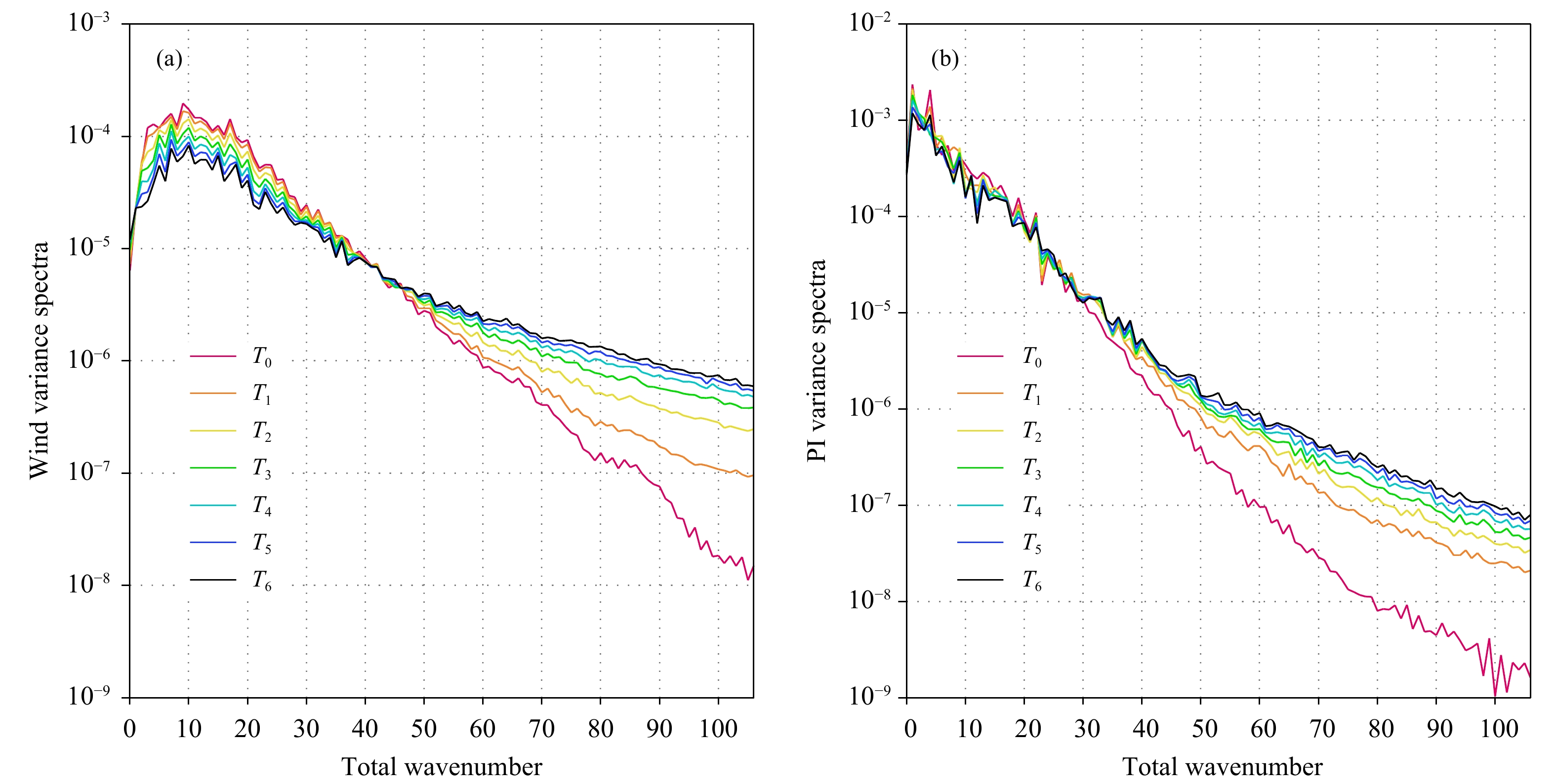图 1  风场 （a，单位：m2/s2）与无量纲气压（b） 分析增量功率谱在6 h同化时间窗的演变 （纵轴为功率谱方差，采用常用对数坐标，T0至T6分别对应同化时间窗起始（${t}_{0}$）至终止（${t}_{{n}}$）时刻） Fig. 1  Variance spectra of analysis increment of vector wind （a，unit：m2/s2） and non-dimensional pressure （b） evolved in the 6 h data assimilation time window （T0 to T6 denote times from the beginning （${t}_{0}$） to the end （${t}_{{n}}$） of the assimilation window）
3 同化时间窗功率谱演变特征诊断 3.1 集合资料同化生成扰动样本

 $\begin{split} J\left({{\text δ}{{\tilde{ X}}^{\rm{a}}}} \right) =& \frac{\,1\,}{\,2\,}{\text δ}{\tilde{ X}^{\rm{aT}}}{{B}}_0^{ - 1}{ \text δ}{\tilde{ X}^{\rm{a}}} + \frac{\,1\,}{\,2\,}\mathop \sum \limits_{{{i}} = 0}^{{n}} {\Big[\left({{{\tilde{ d}}_{{i}}} - {{H}_{{i}}}{{M}_{0,{{i}}}}{\text δ}{{\tilde{ X}}^{\rm{a}}}} \right)^{\rm{T}}}{\text •}\\&{{R}^{ - 1}}\left({{{\tilde{ d}}_{{i}}} - {{H}_{{i}}}{{M}_{0,{{i}}}}{\text δ}{{\tilde{ X}}^{\rm{a}}}} \right)\Big]\\[-12pt]\end{split}$ (9)
 ${{\tilde{{X}}}}^{\mathrm{b}}={\mathcal{M}}_{0,{n}}\left({\tilde{{X}}}_{0}^{\mathrm{a}}\right)+\tilde{{q}}$ (10)

3.2 集合资料同化在GRAPES中的具体实现

GRAPES全球4DVar所使用的观测资料包括探空、小球测风、地面、船舶、飞机、云导风等常规资料，以及欧美微波温度垂直探测资料AMSU-A、红外高光谱垂直探测资料IASI与AIRS、GPS掩星折射率资料等（龚建东等，2016Zhang，et al，2018）。对每种资料的每个观测值按照所给定观测误差叠加高斯型随机扰动，并假定观测之间误差不相关，也即不同观测之间的随机扰动不存在依赖关系。式（9）在具体实现时，选择质量控制后的观测资料，按照集合资料同化的样本数量，对每个观测叠加随机扰动值，生成观测数量相同、观测数值不同的多组观测，每个集合资料同化样本使用其中的一组观测资料。由于相对湿度观测有非负和不能超饱和限制，在叠加高斯型随机扰动时，当相对湿度扰动后的数值小于5%时设为5%，而当出现超饱和时设最大观测为100%。为节省计算量，EDA使用低分辨率全球四维变分同化系统，其外循环分辨率与GRAPES全球4DVar的内循环分辨率一致。EDA外循环分辨率为1.0°，内循环分辨率为1.5°，垂直60层。EDA为逐6 h循环分析系统，每次循环生成40个集合预报样本，统计时总计使用了240个样本。

3.3 误差水平相关的谱特征分析

 $\rho \left(\theta \right) = \frac{{f\left(\theta \right)}}{{f\left(0 \right)}} = \frac{1}{{f\left(0 \right)}}{ \sum \limits_{{n}}}_{}\;{ \sqrt {2{{n}} + 1} \delta _{{n}}^2Y_{{n}}^0}$ (11)

 $f\left(\mathrm{n}\right)={\mathrm{c}\mathrm{o}\mathrm{s}}^{2}\left(\frac{\ {\text{π} }\ }{2}\mathrm{min}\left({n},{{N}}_{\mathrm{t}\mathrm{r}\mathrm{u}\mathrm{n}\mathrm{c}}\right)/{{N}}_{\mathrm{t}\mathrm{r}\mathrm{u}\mathrm{n}\mathrm{c}}\right)$ (12)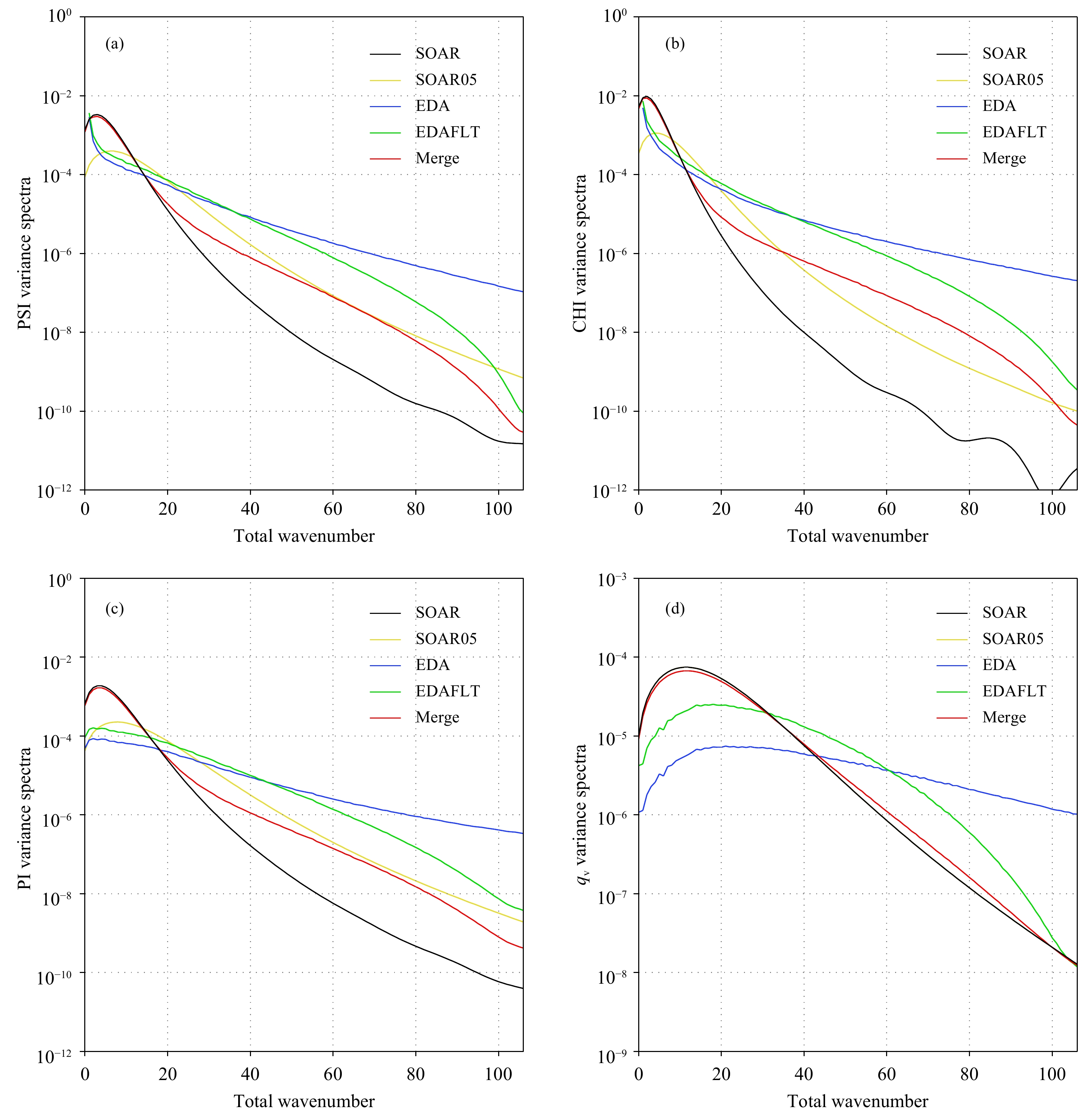图 2  流函数 （a）、势函数 （b）、无量纲气压 （c） 与比湿 （d） 在500 hPa高度的水平相关的功率谱方差特征 （SOAR为二阶自回归相关函数，SOAR05为水平特征长度缩小50%，EDA为集合资料同化样本估计值，EDAFLT为EDA滤波后的估计值，Merge为融合后的数值） Fig. 2  Variance spectra of horizontal correlation for stream-function （a），velocity potential function （b），non-dimensional pressure （c） and specific humidity （d） at 500 hPa geopotential height （SOAR for second-order auto-regression function，SOAR05 for de-correlation length scale reducing 50%，EDA for ensemble member estimation，EDAFLT for EDA with spectral filter，Merge for blending variance spectra）

4 谱空间误差融合对分析的影响 4.1 谱空间误差融合

4.2 误差融合对分析场的影响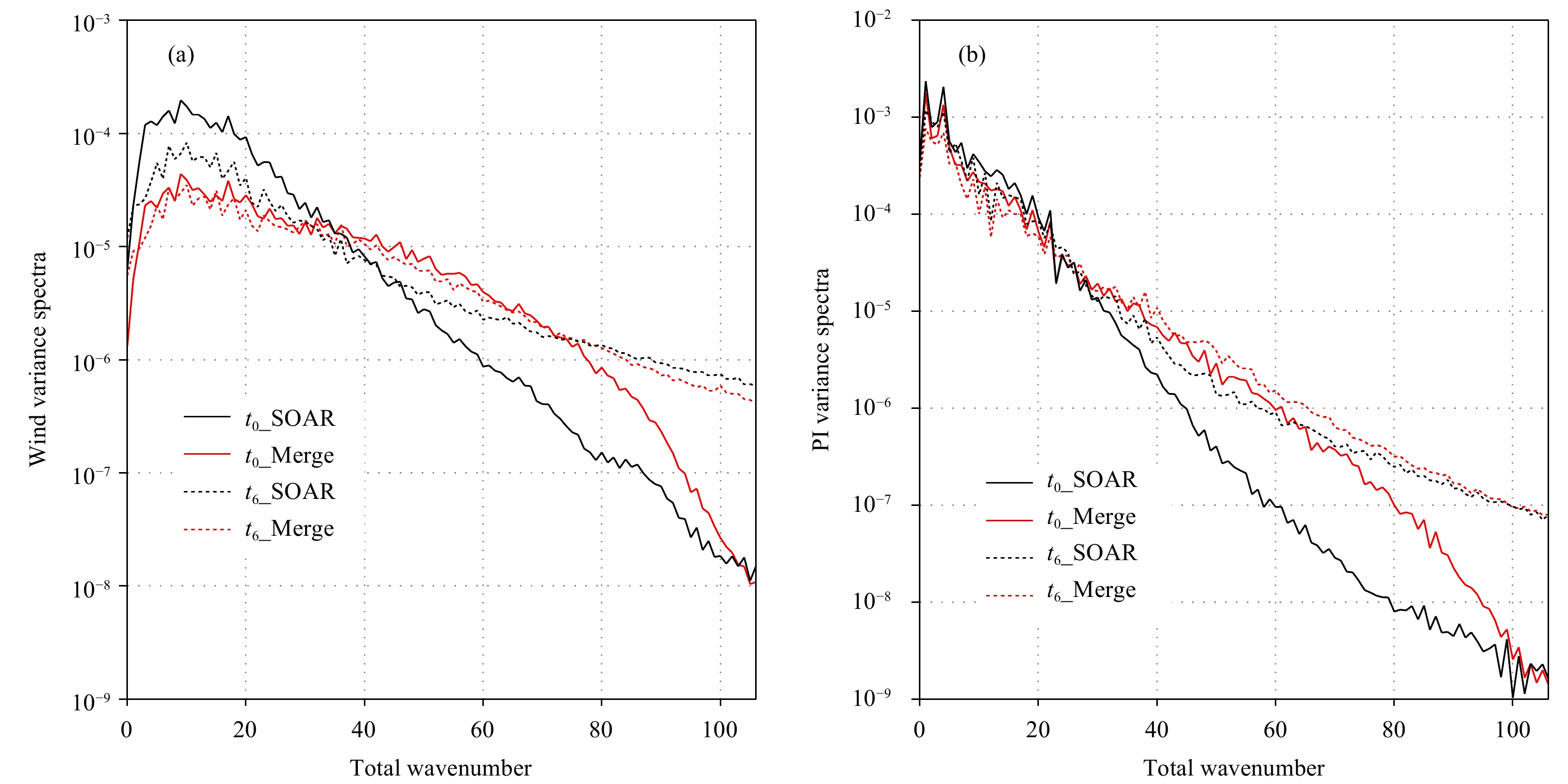图 3  风场（a，单位： ${\mathrm{m}}^{2}/{\mathrm{s}}^{2}$ ）与无量纲气压（b）的分析增量功率谱方差在6 h同化时间窗起始时刻（t0）与终止时刻（tn）的变化 Fig. 3  Variance spectra of analysis increment for vector wind （a，unit： ${\mathrm{m}}^{2}/{\mathrm{s}}^{2}$ ） and non-dimensional pressure （b） evolved at the beginning （t0） and ending （tn） of the 6 h time window

4.3 单点分析增量在格点空间特征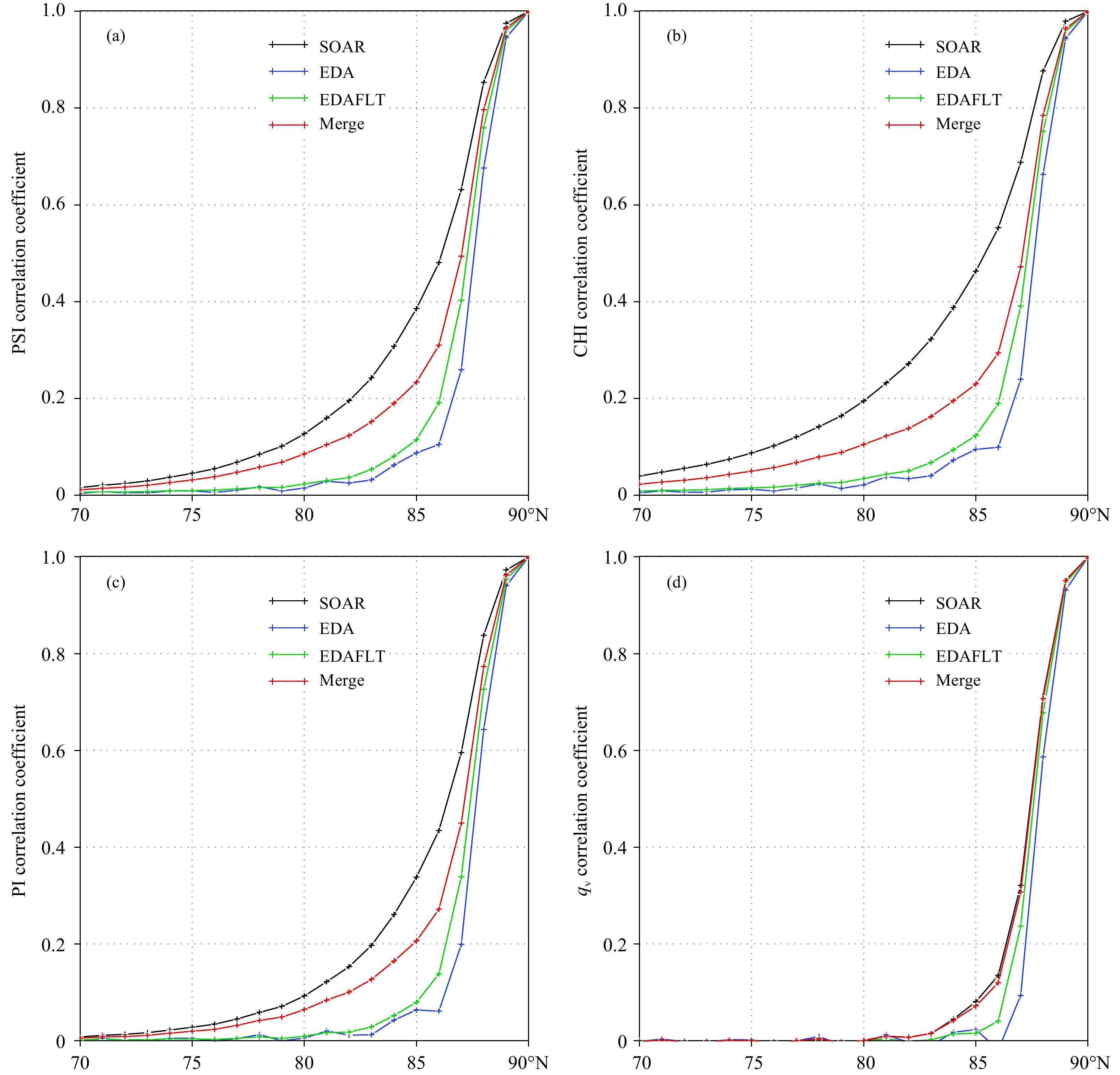图 4  流函数 （a）、势函数 （b）、无量纲气压 （c） 与比湿 （d） 的水平相关系数分布 Fig. 4  Horizontal correlation of stream-function （a），velocity potential-function （b），no-dimensional pressure （c） and specific humidity （d）
4.4 格点空间的分析增量图 5  起始时刻 （t0） 水平风场 （a、b. 单位：m/s） 与温度 （c、d. 单位：K） 的分析增量 （a、c. SOAR水平相关模型，b、d. 融合水平相关） Fig. 5  Horizontal wind （a，b. unit：m/s） and temperature （c，d. unit：K） analysis increment at the beginning time ${t}_{0}$ （a，c. analysis with SOAR；b，d. analysis with blending variance spectra）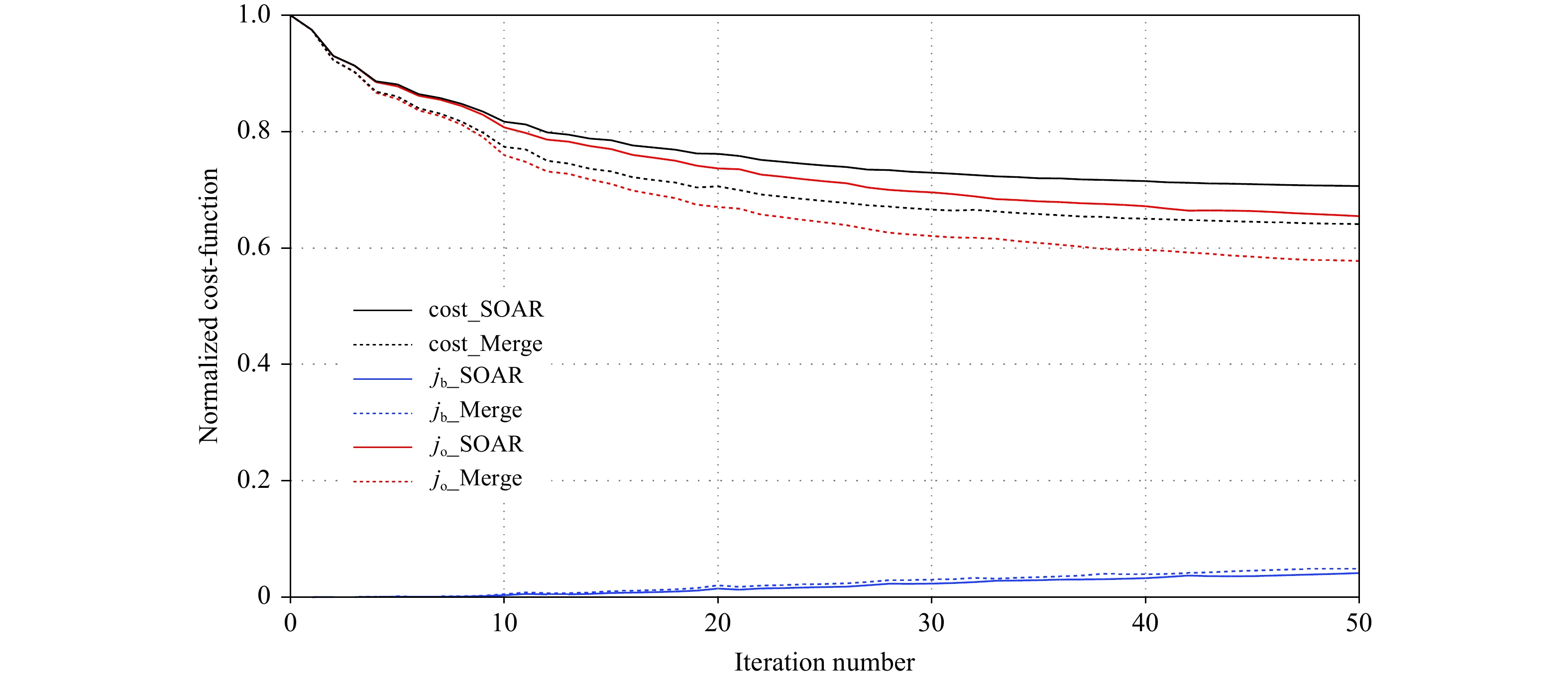图 6  归一化后的目标函数随迭代步数下降的收敛情况 Fig. 6  Converge of normalized cost-function with iteration steps of minimization
5 融合水平相关对分析预报的影响 5.1 对分析与背景误差的影响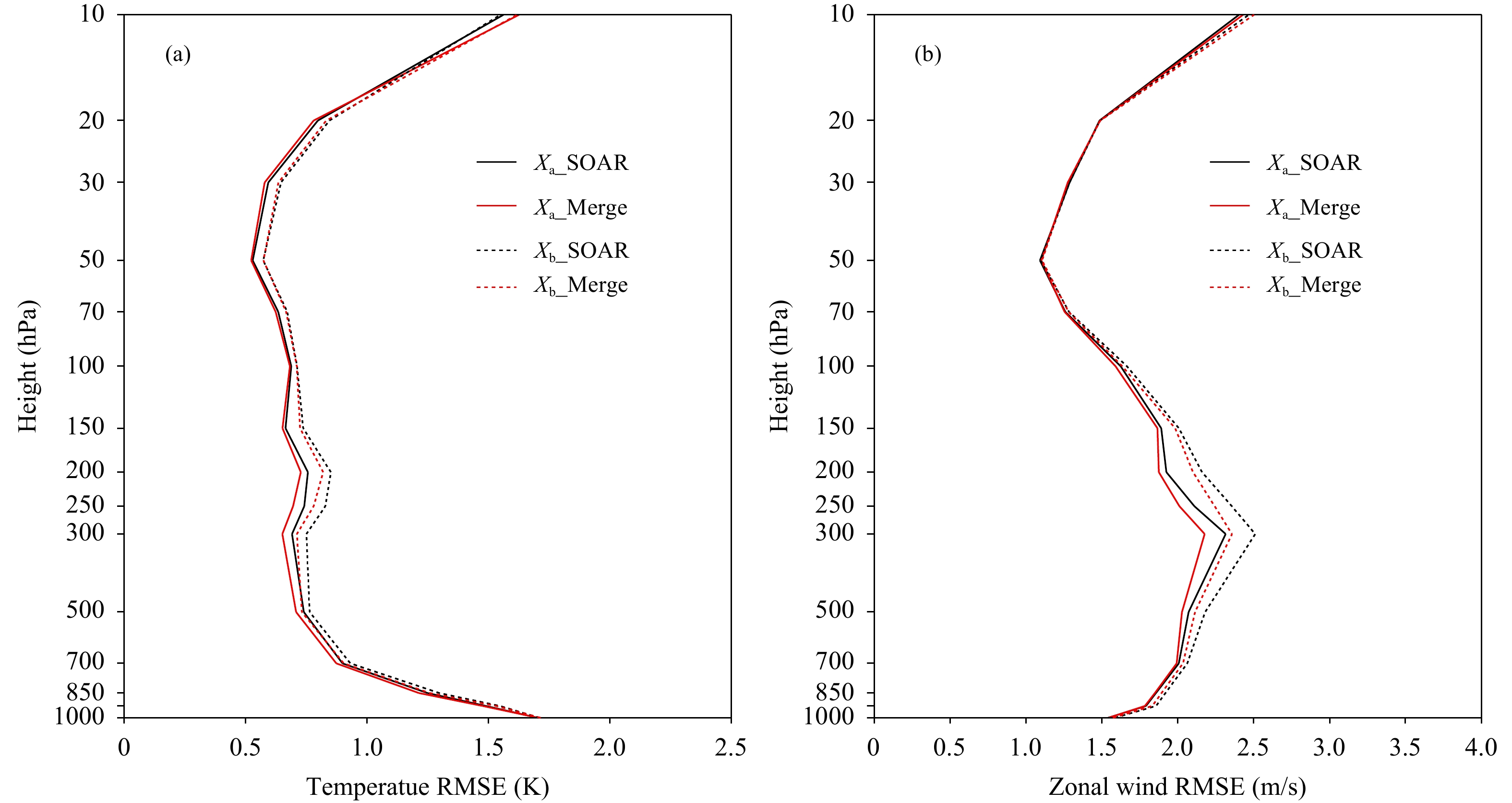图 7  温度 （a） 与东西风场 （b） 的6 h背景场与分析相对于ECMWF再分析的均方根误差垂直分布特征（实线为分析误差，虚线为背景误差；黑线为采用SOAR模型的结果，红线为采用融合相关模型的结果） Fig. 7  Vertical distributions of 6 h background and analysis RMSEs of temperature （a） and zonal wind （b） against ECMWF ERA-Interim reanalysis （The solid-line indicates analysis error，the dashed-line is for background error，the black line denotes results from SOAR，and the red line is for results from blending variance spectra）
5.2 对预报的影响图 8  500 hPa 10 d预报效果 （a、b. 北半球与南半球距平相关系数，c、d. 北半球温度 （单位: K） 与风场 （单位：m/s） 的均方根误差；4DVar1为对照试验，4DVar2为对比试验；两个方案评分差异落在红色方框外代表通过95%的信度检验） Fig. 8  500 hPa 10 d forecast （a，b. ACC of north-hemisphere and south-hemisphere；c，d. RMSE of temperature （unit：K） and vector wind （unit: m/s） of north-hemisphere；4Dvar1 for control experiment，4DVar2 for comparative experiment；differences outside the hallow bars are significant at the 95% confidence level）
6 总结与讨论

Järvinen（2001）研究表明，在观测空间的6 h同化时间窗随着预报时效延长背景误差水平相关特征呈现出逐渐增大的特征，其原因与背景误差随切线模式的时间积分出现误差能量跨尺度的传播，从小尺度向更大尺度波传播有关。本研究通过融合水平相关模型改善了原有相关模型在50波以上的功率谱在终止时刻要明显大于起始时刻，在同化时间窗内则存在明显不一致的不足，但没有发现从小尺度向更大尺度波传播的特征，这一点在未来的研究中还需要进一步诊断误差能量的传播特征来更合理地表述背景误差协方差的演变。

 龚建东. 2007. 资料同化中二维特征长度随模式分辨率变化的分析研究. 大气科学, 31(3): 459-467. Gong J D. 2007. The analysis on variation of horizontal de-correlation length with model resolution in data assimilation system. Chinese J Atmos Sci, 31(3): 459-467. (in Chinese) 龚建东, 王瑞春, 郝民. 2016. 温湿统计平衡约束关系对GRAPES全球湿度分析的作用. 气象学报, 74(3): 380-396. Gong J D, Wang R C, Hao M. 2016. The impact of a balance constraint between temperature and humidity on the global humidity analysis in GRAPES. Acta Meteor Sinica, 74(3): 380-396. DOI:10.11676/qxxb2016.035 (in Chinese) 龚建东, 刘永柱, 张林. 2019. 面向四维变分资料同化的NSAS积云深对流参数化方案的简化及线性化研究. 气象学报, 77(4): 595-616. Gong J D, Liu Y Z, Zhang L. 2019. A study of simplification and linearization of the NSAS deep convection cumulus parameterization scheme for 4D-Var. Acta Meteor Sinica, 77(4): 595-616. DOI:10.11676/qxxb2019.048 (in Chinese) 刘艳, 薛纪善. 2019. GRAPES的新初始化方案. 气象学报, 77(2): 165-179. Liu Y, Xue J S. 2019. The new initialization scheme of the GRAPES. Acta Meteor Sinica, 77(2): 165-179. DOI:10.11676/qxxb2019.029 (in Chinese) 刘永柱, 张林, 金之雁. 2017. GRAPES全球切线性和伴随模式的调优. 应用气象学报, 28(1): 62-71. Liu Y Z, Zhang L, Jin Z Y. 2017. The optimization of GRAPES global tangent linear model and adjoint model. J Appl Meteor Sci, 28(1): 62-71. DOI:10.11898/1001-7313.20170106 (in Chinese) 王金成, 庄照荣, 韩威等. 2014. GRAPES全球变分同化背景误差协方差的改进及对分析预报的影响: 背景误差协方差三维结构的估计. 气象学报, 72(1): 62-78. Wang J C, Zhuang Z R, Han W, et al. 2014. An improvement of background error covariance in the global GRAPES variational data assimilation and its impact on the analysis and prediction: Statistics of the three-dimensional structure of background error covariance. Acta Meteor Sinica, 72(1): 62-78. DOI:10.11676/qxxb2014.008 (in Chinese) 王瑞春, 龚建东, 张林等. 2015. 热带风压场平衡特征及其对GRAPES系统中同化预报的影响研究 II: 动力与统计混合平衡约束方案的应用. 大气科学, 39(6): 1225-1236. Wang R C, Gong J D, Zhang L, et al. 2015. Tropical balance characteristics between mass and wind fields and their impact on analyses and forecasts in GRAPES system. Part II: Application of linear balance equation-regression hybrid constraint scheme. Chinese J Atmos Sci, 39(6): 1225-1236. (in Chinese) 薛纪善. 2009. 气象卫星资料同化的科学问题与前景. 气象学报, 67(6): 903-911. Xue J S. 2009. Scientific issues and perspective of assimilation of meteorological satellite data. Acta Meteor Sinica, 67(6): 903-911. DOI:10.11676/qxxb2009.088 (in Chinese) 张华, 薛纪善, 庄世宇等. 2004. GRAPES三维变分同化系统的理想试验. 气象学报, 62(1): 31-41. Zhang H, Xue J S, Zhuang S X, et al. 2004. Idea experiments of GRAPES three-dimensional variational data assimilation system. Acta Meteor Sinica, 62(1): 31-41. DOI:10.11676/qxxb2004.004 (in Chinese) 张林, 刘永柱. 2017. GRAPES全球四维变分同化系统极小化算法预调节. 应用气象学报, 28(2): 168-176. Zhang L, Liu Y Z. 2017. The preconditioning of minimization algorithm in GRAPES global four-dimensional variational data assimilation system. J Appl Meteor Sci, 28(2): 168-176. DOI:10.11898/1001-7313.20170204 (in Chinese) Bannister R N. 2008a. A review of forecast error covariance statistics in atmospheric variational data assimilation. I: Characteristics and measurements of forecast error covariances. Quart J Roy Meteor Soc, 134(637): 1951-1970. DOI:10.1002/qj.339 Bannister R N. 2008b. A review of forecast error covariance statistics in atmospheric variational data assimilation. II: Modelling the forecast error covariance statistics. Quart J Roy Meteor Soc, 134(637): 1971-1996. DOI:10.1002/qj.340 Boer G J, Shepherd T G. 1983. Large-scale two-dimensional turbulence in the atmosphere. J Atmos Sci, 40(1): 164-184. DOI:10.1175/1520-0469(1983)040<0164:LSTDTI>2.0.CO;2 Bonavita M, Raynaud L, Isaksen L. 2011. Estimating background-error variances with the ECMWF Ensemble of Data Assimilations system: Some effects of ensemble size and day-to-day variability. Quart J Roy Meteor Soc, 137(655): 423-434. DOI:10.1002/qj.756 Daley R. 1991. Atmospheric Data Analysis. Cambridge: Cambridge University Press, 457pp Derber J, Bouttier F. 1999. A reformulation of the background error covariance in the ECMWF global data assimilation system. Tellus A, 51(2): 195-221. DOI:10.3402/tellusa.v51i2.12316 Fisher M. 2003. Background error covariance modelling∥Proceedings of the ECMWF Seminar on Recent Developments in Data Assimilation for Atmosphere and Ocean. Shinfield Park, Reading: ECMWF Gauthier P, Thépaut J N. 2001. Impact of the digital filter as a weak constraint in the preoperational 4DVar assimilation system of Météo-France. Mon Wea Rev, 129(8): 2089-2102. DOI:10.1175/1520-0493(2001)129<2089:IOTDFA>2.0.CO;2 Gauthier P, Tanguay M, Laroche S, et al. 2007. Extension of 3DVar to 4DVar: Implementation of 4DVar at the meteorological service of Canada. Mon Wea Rev, 135(6): 2339-2354. DOI:10.1175/MWR3394.1 Isaksen L, Bonavita M, Buizza R, et al. 2010. Ensemble of data assimilations at ECMWF. ECMWF Technical Memorandum 636 Järvinen H. 2001. Temporal evolution of innovation and residual statistics in the ECMWF variational data assimilation systems. Tellus A, 53(3): 333-347. DOI:10.3402/tellusa.v53i3.12192 Lopez P, Moreau E. 2005. A convection scheme for data assimilation: Description and initial tests. Quart J Roy Meteor Soc, 131(606): 409-436. DOI:10.1256/qj.04.69 Lorenc A C, Rawlins F. 2005. Why does 4D-Var beat 3D-Var?. Quart J Roy Meteor Soc, 131（613）: 3247-3257 Maturi E, Harris A, Mittaz J, et al. 2017. A new high-resolution sea surface temperature blended analysis. Bull Amer Meteor Soc, 98(5): 1015-1026. DOI:10.1175/BAMS-D-15-00002.1 Purser R J, Wu W S, Parrish D F, et al. 2003. Numerical aspects of the application of recursive filters to variational statistical analysis. Part II: Spatially inhomogeneous and anisotropic general covariances. Mon Wea Rev, 131(8): 1536-1548. DOI:10.1175//2543.1 Rabier F, Järvinen H, Klinker E, et al. 2000. The ECMWF operational implementation of four-dimensional variational assimilation. I: Experimental results with simplified physics. Quart J Roy Meteor Soc, 126(564): 1143-1170. DOI:10.1002/qj.49712656415 Rawlins F, Ballard S P, Bovis K J, et al. 2007. The Met Office global four-dimensional variational data assimilation scheme. Quart J Roy Meteor Soc, 133(623): 347-362. DOI:10.1002/qj.32 Raynaud L, Berre L, Desroziers G. 2009. Objective filtering of ensemble-based background-error variances. Quart J Roy Meteor Soc, 135(642): 1177-1199. DOI:10.1002/qj.438 Reynolds R W, Smith T M, Liu C Y, et al. 2007. Daily high-resolution-blended analyses for sea surface temperature. J Climate, 20(22): 5473-5496. DOI:10.1175/2007JCLI1824.1 Talagrand O, Courtier P. 1987. Variational assimilation of meteorological observations with the adjoint vorticity equation. I: Theory. Quart J Roy Meteor Soc, 113(478): 1311-1328. DOI:10.1002/qj.49711347812 Wee T K, Kuo Y H. 2004. Impact of a digital filter as a weak constraint in MM5 4DVar: An observing system simulation experiment. Mon Wea Rev, 132(2): 543-559. DOI:10.1175/1520-0493(2004)132<0543:IOADFA>2.0.CO;2 Zhang L, Liu Y Z, Liu Y, et al. 2019. The operational global four-dimensional variational data assimilation system at the China Meteorological Administration. Quart J Roy Meteor Soc, 145(722): 1882-1896. DOI:10.1002/qj.3533 Zhang L H, Gong J D, Wang R C. 2018. Diagnostic analysis of various observation impacts in the 3DVar assimilation system of global GRAPES. Mon Wea Rev, 146(10): 3125-3142. DOI:10.1175/MWR-D-17-0182.1